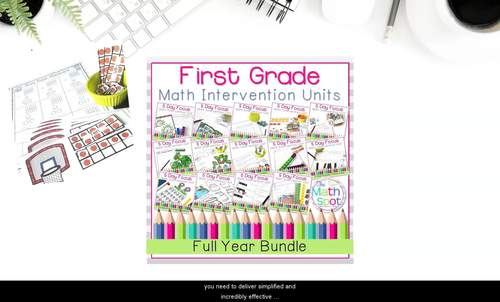# First Grade Math Intervention | Lesson BundleSubject
Resource Type
File Type

Zip

(66 MB|500 + )
Standards
15 Products in this Bundle
15 products
Also included in:
1. This amazing first grade math intervention bundle has you covered for the entire year! This bundle includes all materials a math interventionist would need to run successful small group lessons for a school year in order to remediate 1st grade math skills and standards. This Bundle Contains 4 Main C
\$196.98
\$150.00
Save \$46.98
• Bundle Description
• Standards

This bundle of 1st grade math intervention units supports you in delivering quality math intervention, RtI and progress monitoring for your first grade small groups.

Each unit includes:

• A Pre and Post Unit Assessment
• 5 Detailed Daily Lessons
• Independent Practice Activities
• Daily Ticket Out The Door Assessments

How Does 5 Day Focus Math Intervention Work?

This full-year curriculum bundle includes 14 units designed for small group math instruction. These units will make planning effective instruction so much easier because they include everything you need to instruct, assess, and provide independent practice to your students.

• Each unit begins with an introduction that gives you a clear understanding of the unit to come and the strategies that will be used. I keep the unit introduction short and sweet while orienting you to the standards you are addressing in the unit.
• Next, you will find a unit overview that gives you an at-a-glance peek at the lesson topics, independent activities, and assessment. Stay organized easily with the overview!
• For each unit, you will find a pre and post assessment along with daily exit tickets so that you can monitor your students' progress towards the unit goals. These assessments are ideal to have in your back pocket come time for report cards, progress reports, or student meetings.
• Next, each unit includes 5 lessons. These lessons are based on the concrete -> representational -> abstract progression and include activities that will support your students in understanding these tricky math concepts.
• Last, but not least... in fact, I think this may be the MOST important component.... each lesson comes with an independent practice activity so that you can be sure that your students will leave each unit independently able to demonstrate the target skill. No longer will you say "they could do it in the group but independently they fall apart". We build this in because the goal is independence in each and every unit.

Included Units:

Building a Foundation

Numbers & Operations to 20

Numbers & Operations to 100

Determine the unknown whole number in an addition or subtraction equation relating three whole numbers. For example, determine the unknown number that makes the equation true in each of the equations 8 + ? = 11, 5 = ▯ - 3, 6 + 6 = ▯.
Understand the meaning of the equal sign, and determine if equations involving addition and subtraction are true or false. For example, which of the following equations are true and which are false? 6 = 6, 7 = 8 - 1, 5 + 2 = 2 + 5, 4 + 1 = 5 + 2.
Add and subtract within 20, demonstrating fluency for addition and subtraction within 10. Use strategies such as counting on; making ten (e.g., 8 + 6 = 8 + 2 + 4 = 10 + 4 = 14); decomposing a number leading to a ten (e.g., 13 - 4 = 13 - 3 - 1 = 10 - 1 = 9); using the relationship between addition and subtraction (e.g., knowing that 8 + 4 = 12, one knows 12 - 8 = 4); and creating equivalent but easier or known sums (e.g., adding 6 + 7 by creating the known equivalent 6 + 6 + 1 = 12 + 1 = 13).
Relate counting to addition and subtraction (e.g., by counting on 2 to add 2).
Understand subtraction as an unknown-addend problem. For example, subtract 10 – 8 by finding the number that makes 10 when added to 8.
Total Pages
500 +
N/A
Teaching Duration
N/A
Report this Resource to TpT
Reported resources will be reviewed by our team. Report this resource to let us know if this resource violates TpT’s content guidelines.\$90.00
Bundle
List Price:
\$112.00
You Save:
\$22.00